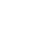447 views
447
i like this count better. it seems to have the right look. waves a & b are complete. wave c is underway; ( iii ) possibly extending. target is 320 for the end of wave c/((v))johnrfraser
i don't know where exactly is the end of wave c/((v)). the market did manage to go beyond the minimum target which was 287. so, anywhere between the minimum and 320 could mark the end of wave a of 5. we can use macd to help us identify wave three to calculate where wave five will end